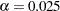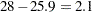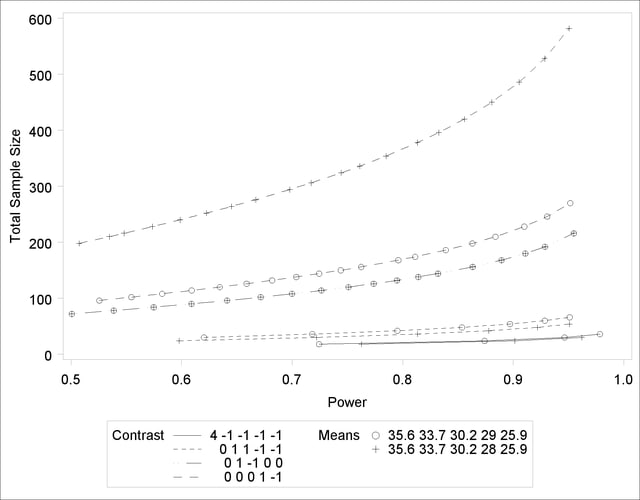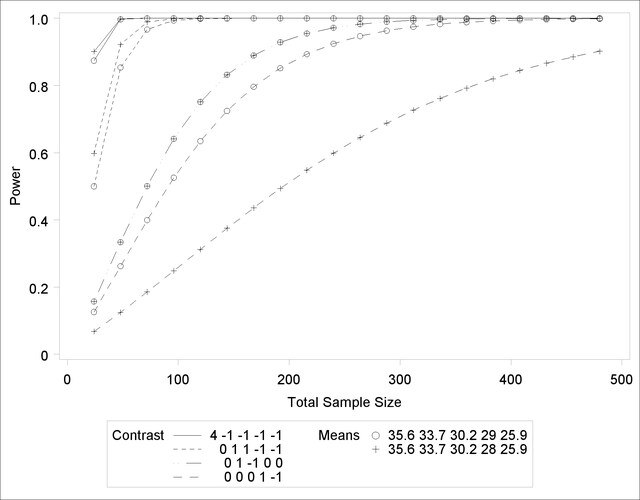Previous Page | Next Page

 The POWER Procedure

Example 68.1 One-Way ANOVA

This example deals with the same situation as in Example 41.1 of Chapter 41, The GLMPOWER Procedure.

Hocking (1985, p. 109) describes a study of the effectiveness of electrolytes in reducing lactic acid buildup for long-distance runners. You are planning a similar study in which you will allocate five different fluids to runners on a 10-mile course and measure lactic acid buildup immediately after the run. The fluids consist of water and two commercial electrolyte drinks, EZDure and LactoZap, each prepared at two concentrations, low (EZD1 and LZ1) and high (EZD2 and LZ2).

You conjecture that the standard deviation of lactic acid measurements given any particular fluid is about 3.75, and that the expected lactic acid values will correspond roughly to those in Table 68.31. You are least familiar with the LZ1 drink and hence decide to consider a range of reasonable values for that mean.

 Water EZD1 EZD2 LZ1 LZ2 35.6 33.7 30.2 29 or 28 25.9

You are interested in four different comparisons, shown in Table 68.32 with appropriate contrast coefficients.

 Contrast Coefficients Comparison Water EZD1 EZD2 LZ1 LZ2 Water versus electrolytes 4 –1 –1 –1 –1 EZD versus LZ 0 1 1 –1 –1 EZD1 versus EZD2 0 1 –1 0 0 LZ1 versus LZ2 0 0 0 1 –1

For each of these contrasts you want to determine the sample size required to achieve a power of 0.9 for detecting an effect with magnitude in accord with Table 68.31. You are not yet attempting to choose a single sample size for the study, but rather checking the range of sample sizes needed for individual contrasts. You plan to test each contrast at. In the interests of reducing costs, you will provide twice as many runners with water as with any of the electrolytes; in other words, you will use a sample size weighting scheme of 2:1:1:1:1. Use the ONEWAYANOVA statement in the POWER procedure to compute the sample sizes.

The statements required to perform this analysis are as follows:

```proc power;
onewayanova
groupmeans = 35.6 | 33.7 | 30.2 | 29 28 | 25.9
stddev = 3.75
groupweights = (2 1 1 1 1)
alpha = 0.025
ntotal = .
power = 0.9
contrast = (4 -1 -1 -1 -1) (0  1  1 -1 -1)
(0  1 -1  0  0) (0  0  0  1 -1);
run;
```

The NTOTAL= option with a missing value (.) indicates total sample size as the result parameter. The GROUPMEANS= option with values from Table 68.31 specifies your conjectures for the means. With only one mean varying (the LZ1 mean), the "crossed" notation is simpler, showing scenarios for each group mean, separated by vertical bars (|). See the section Specifying Value Lists in Analysis Statements for more details on crossed and matched notations for grouped values. The contrasts in Table 68.32 are specified with the CONTRAST= option, by using the "matched" notation with each contrast enclosed in parentheses. The STDDEV=, ALPHA=, and POWER= options specify the error standard deviation, significance level, and power. The GROUPWEIGHTS= option specifies the weighting schemes. Default values for the NULLCONTRAST= and SIDES= options specify a two-sidedtest of the contrast equal to 0. See Output 68.1.1 for the results.

Output 68.1.1 Sample Sizes for One-Way ANOVA Contrasts
The POWER Procedure
Single DF Contrast in One-Way ANOVA

Fixed Scenario Elements
Method Exact
Alpha 0.025
Standard Deviation 3.75
Group Weights 2 1 1 1 1
Nominal Power 0.9
Number of Sides 2
Null Contrast Value 0

Computed N Total
Index Contrast Means Actual Power N Total
1 4 -1 -1 -1 -1 35.6 33.7 30.2 29 25.9 0.947 30
2 4 -1 -1 -1 -1 35.6 33.7 30.2 28 25.9 0.901 24
3 0 1 1 -1 -1 35.6 33.7 30.2 29 25.9 0.929 60
4 0 1 1 -1 -1 35.6 33.7 30.2 28 25.9 0.922 48
5 0 1 -1 0 0 35.6 33.7 30.2 29 25.9 0.901 174
6 0 1 -1 0 0 35.6 33.7 30.2 28 25.9 0.901 174
7 0 0 0 1 -1 35.6 33.7 30.2 29 25.9 0.902 222
8 0 0 0 1 -1 35.6 33.7 30.2 28 25.9 0.902 480

The sample sizes in Output 68.1.1 range from 24 for the comparison of water versus electrolytes to 480 for the comparison of LZ1 versus LZ2, both assuming the smaller LZ1 mean. The sample size for the latter comparison is relatively large because the small mean difference ofis hard to detect.

The Nominal Power of 0.9 in the "Fixed Scenario Elements" table in Output 68.1.1 represents the input target power, and the Actual Power column in the "Computed N Total" table is the power at the sample size (N Total) adjusted to achieve the specified sample weighting. Note that all of the sample sizes are rounded up to multiples of 6 to preserve integer group sizes (since the group weights add up to 6). You can use the NFRACTIONAL option in the ONEWAYANOVA statement to compute raw fractional sample sizes.

Suppose you want to plot the required sample size for the range of power values from 0.5 to 0.95. First, define the analysis by specifying the same statements as before, but add the PLOTONLY option to the PROC POWER statement to disable the nongraphical results. Next, specify the PLOT statement with X=POWER to request a plot with power on the X axis. (The result parameter, here sample size, is always plotted on the other axis.) Use the MIN= and MAX= options in the PLOT statement to specify the power range. The following statements produce the plot shown in Output 68.1.2.

```proc power plotonly;
onewayanova
groupmeans = 35.6 | 33.7 | 30.2 | 29 28 | 25.9
stddev = 3.75
groupweights = (2 1 1 1 1)
alpha = 0.025
ntotal = .
power = 0.9
contrast = (4 -1 -1 -1 -1) (0  1  1 -1 -1)
(0  1 -1  0  0) (0  0  0  1 -1);
plot x=power min=.5 max=.95;
run;
```

Output 68.1.2 Plot of Sample Size versus Power for One-Way ANOVA ContrastsIn Output 68.1.2, the line style identifies the contrast, and the plotting symbol identifies the group means scenario. The plot shows that the required sample size is highest for the (0 0 0 1 –1) contrast, corresponding to the test of LZ1 versus LZ2 that was previously found to require the most resources, in either cell means scenario.

Note that some of the plotted points in Output 68.1.2 are unevenly spaced. This is because the plotted points are the rounded sample size results at their corresponding actual power levels. The range specified with the MIN= and MAX= values in the PLOT statement corresponds to nominal power levels. In some cases, actual power is substantially higher than nominal power. To obtain plots with evenly spaced points (but with fractional sample sizes at the computed points), you can use the NFRACTIONAL option in the analysis statement preceding the PLOT statement.

Finally, suppose you want to plot the power for the range of sample sizes you will likely consider for the study (the range of 24 to 480 that achieves 0.9 power for different comparisons). In the ONEWAYANOVA statement, identify power as the result (POWER=.), and specify NTOTAL=24. The following statements produce the plot:

```proc power plotonly;
onewayanova
groupmeans = 35.6 | 33.7 | 30.2 | 29 28 | 25.9
stddev = 3.75
groupweights = (2 1 1 1 1)
alpha = 0.025
ntotal = 24
power = .
contrast = (4 -1 -1 -1 -1) (0  1  1 -1 -1)
(0  1 -1  0  0) (0  0  0  1 -1);
plot x=n min=24 max=480;
run;
```

The X=N option in the PLOT statement requests a plot with sample size on the X axis.

Note that the value specified with the NTOTAL=24 option is not used. It is overridden in the plot by the MIN= and MAX= options in the PLOT statement, and the PLOTONLY option in the PROC POWER statement disables nongraphical results. But the NTOTAL= option (along with a value) is still needed in the ONEWAYANOVA statement as a placeholder, to identify the desired parameterization for sample size.

Output 68.1.3 shows the resulting plot.

Output 68.1.3 Plot of Power versus Sample Size for One-Way ANOVA ContrastsAlthough Output 68.1.2 and Output 68.1.3 surface essentially the same computations for practical power ranges, they each provide a different quick visual assessment. Output 68.1.2 reveals the range of required sample sizes for powers of interest, and Output 68.1.3 reveals the range of achieved powers for sample sizes of interest.Previous Page | Next Page | Top of Page